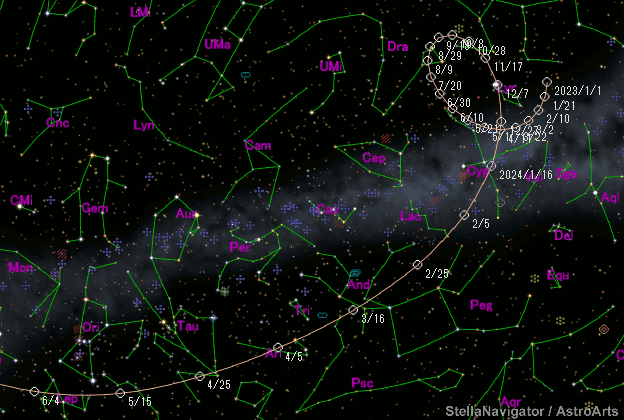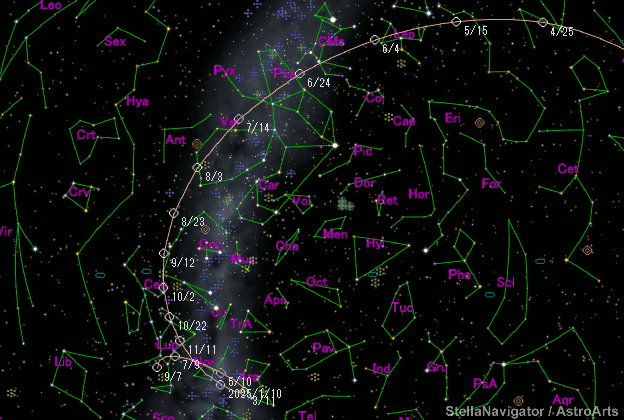# \$B%]%s%9(B-\$B%V%k%C%/%9WB@1(B

12P/Pons-Brooks (2024)###\$B50F;MWAG(B

```Perturbed ephemeris below is based on elements from QJ 27, 604.

Epoch = 2460440.50000 TT   T = 2460421.82800 TT
Peri. =  198.98996         a =    17.1988963 AU
Node  =  255.85632 J2000.0 e =     0.9546027
Incl. =   74.19160         q =     0.7807832 AU
```

###\$B@1?^(B###\$B8wEYJQ2=(B

```        m1 = 5.0 + 5 log\$B&\$(B + 15.0 log r
```##### \$B50F;MWAG\$O(BMinor Planet & Comet Ephemeris Service\$B\$G7W;;\$7\$?\$b\$N\$G\$9!#(B \$B@1?^\$O%9%F%i%J%S%2!<%?(B Ver.10 (\$B%"%9%H%m%"!<%D(B) \$B\$G:n@.\$7\$?\$b\$N\$G\$9!#(B \$B8wEY%0%i%U\$O(BComet for Windows\$B\$G:n@.\$7\$?\$b\$N\$G\$9!#(B